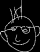# Courses

## Spring 2001

Mathematics 309 - Topics in Geometry

The main page for Mathematics 103 - Calculus for the Life Sciences

## Fall 2000

Mathematics 220

The main page for Mathematics 102 - Calculus for the Life Sciences

## Spring 2000

Mathematics 266 Vector calculus for Electrical Engineers

The main page for Mathematics 103 - Calculus for the Life Sciences

## Fall 1999

Mathematics 308 Euclidean geometry

The main page for Mathematics 102 - Calculus for the Life Sciences

## Spring 1999

Mathematics 152 Linear algebra for engineers

Mathematics 266 Vector calculus and complex analysis for electrical engineers

Mathematics 431 Special topics in geometry

## Fall 1998

Mathematics 308 Euclidean geometry

Mathematics 256 Differential equations for engineers

Mathematics 100 and Mathematics 101 First year calculus (as taught by David Austin, Leah Keshet, and Denis Sjerve)

## Spring 1998

Mathematics 309 Topics in geometry

## Fall 1997

Mathematics 308 Euclidean geometry

Mathematics 256 Differential equations for engineers

## Spring 1997

Mathematics 152 Linear algebra for engineers

Mathematics 309 Topics in geometry

## Fall 1996

Mathematics 308 Euclidean geometry

Mathematics 256 Differential equations for engineers

## Spring 1996

Mathematics 255 Ordinary differential equations

Mathematics 257 Partial differential equations

Mathematics 308 Euclidean geometry

## Fall 1995

Mathematics 307 Applied linear algebra

## The programming language PostScript

PostScript is an interpreted language originally intended for use in printers. It can be used for many tasks involving complicated graphics, and even for a wide range of general programming purposes. It is not suitable for serious programming endeavours, since it is somewhat slow and it can handle only a very limited range of integers and real numbers, but I believe it is undervalued as an educational tool.

I use PostScript in a number of my courses for various purposes: (1) Mathematics 308, to show how geometry can be used to draw sophisticated mathematical pictures; (2) Mathematics 307, as the base for a convenient linear algebra calculator. I have just begun to construct animated sequences of physical systems, which might serve as prototypes for final versions to be done in Java.

There exist free versions of PostScript interpreters for UNIX, MSDOS, and Macs. They can all be found at the GhostScript home page

## Remarks on student talks in undergraduate classes

Free advice (worth every penny you pay for it) for students and colleagues.